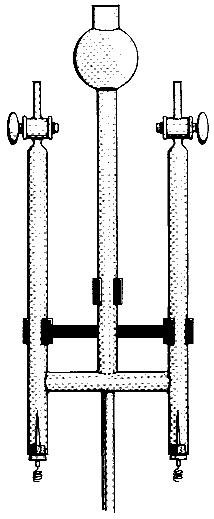# The Electrolysis of Water

$$\newcommand{\vecs}{\overset { \rightharpoonup} {\mathbf{#1}} }$$ $$\newcommand{\vecd}{\overset{-\!-\!\rightharpoonup}{\vphantom{a}\smash {#1}}}$$$$\newcommand{\id}{\mathrm{id}}$$ $$\newcommand{\Span}{\mathrm{span}}$$ $$\newcommand{\kernel}{\mathrm{null}\,}$$ $$\newcommand{\range}{\mathrm{range}\,}$$ $$\newcommand{\RealPart}{\mathrm{Re}}$$ $$\newcommand{\ImaginaryPart}{\mathrm{Im}}$$ $$\newcommand{\Argument}{\mathrm{Arg}}$$ $$\newcommand{\norm}{\| #1 \|}$$ $$\newcommand{\inner}{\langle #1, #2 \rangle}$$ $$\newcommand{\Span}{\mathrm{span}}$$ $$\newcommand{\id}{\mathrm{id}}$$ $$\newcommand{\Span}{\mathrm{span}}$$ $$\newcommand{\kernel}{\mathrm{null}\,}$$ $$\newcommand{\range}{\mathrm{range}\,}$$ $$\newcommand{\RealPart}{\mathrm{Re}}$$ $$\newcommand{\ImaginaryPart}{\mathrm{Im}}$$ $$\newcommand{\Argument}{\mathrm{Arg}}$$ $$\newcommand{\norm}{\| #1 \|}$$ $$\newcommand{\inner}{\langle #1, #2 \rangle}$$ $$\newcommand{\Span}{\mathrm{span}}$$$$\newcommand{\AA}{\unicode[.8,0]{x212B}}$$

## Chemical Concepts Demonstrated

• Electrolysis of water
• Decomposition of water

## Demonstration

The Hoffman electrolysis apparatus is filled with Na2SO4 solution containing universal indicator and is started.## Observations

The solution turns blue at the cathode (basic) and red at the anode (acidic). Twice as much gas is evolved at the cathode as at the anode. When the solutions in the two electrodes compartments are mixed, the indicator turns green (neutral).

## Explanations (including important chemical equations)

Two reactions can take place at the cathode.

 2 H2O (l) + 2 e - → H2 (g) + 2 OH- (aq) E° = -0.8277 V Na + (aq) + e - → Na (s) E° = -2.7109 V

Two reactions can also occur at the anode.

 2 SO4 2- (aq) → S2O8 (aq) + 2 e - E° = -2.05 V 2 H2O (l) → O2(g) + 4 H+ (aq) + 4 e - E° = -1.229 V

It is easier to reduce H2O that Na+ ions at the cathode and it is easier to oxidize H2O than SO4 2- at the anode. Combining the two half reactions so that electrons are conserved, we obtain the following equations.

6 H2O (l) → 2 H2 (g) + O2 (g) + 4 H + (aq) + 4 OH- (aq)

or

2 H2O (l) → 2 H2 (g) + O2(g)

The decomposition of water produces twice as much hydrogen gas as oxygen gas. Since the number of protons and hydroxide ions formed in the reaction are the same, when the solutions are combined at the end of the electrolysis the indicator has the characteristic green color of a neutral solution.

The Electrolysis of Water is shared under a CC BY-NC-SA 4.0 license and was authored, remixed, and/or curated by George Bodner.